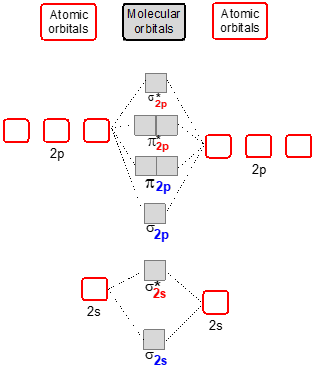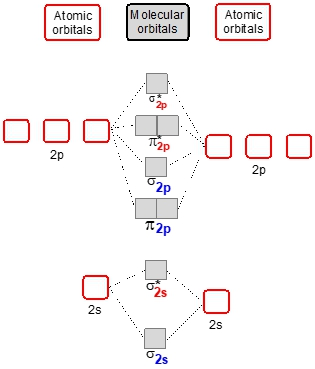## General Chemistry

Learn the toughest concepts covered in Chemistry with step-by-step video tutorials and practice problems by world-class tutors

12. Molecular Shapes & Valence Bond Theory

# MO Theory: Heteronuclear Diatomic Molecules

Heteronuclear Diatomic Molecules are composed of 2 different elements bonded together.

Molecular Orbital Diagrams of Heteronuclear Diatomics
1
concept

## MO Theory: Heteronuclear Diatomic Molecules Concept 12m
Play a video:
recall that a hetero nuclear diatonic molecule is composed of two different elements bonded together. And we're going to say here that the less electro negative element determines which molecular orbital will be used and recall that electro negativity increases as moved to the top right corner Of the periodic table. Remember flooring is the most electro negative element with the value of four point up. Now we're going to say here that when it comes to displaying these molecular orbital's or these molecular orbital diagrams, the more electro negative element within the hetero nuclear di atomic molecules possesses atomic orbital that are lower in energy. So here, if we take a look, we have molecular orbital diagrams for hydrogen to helium for lithium to nitrogen and from oxygen to neon, if you watch my videos on molecular orbital theory, you're kind of familiar with these displays. Now if you notice, you can see here that this two s atomic orbital is higher in energy than this one. This one's lower down. That must mean that let's say we're combining work comparing carbon to nitrogen. Carbon is less electoral negative than nitrogen, since nitrogen is more electro negative, it would be here on this side and carbon would be here on this side and since nitrogen is more electro negative, that explains why its atomic orbital are lower in energy. See here, it's two p orbital czar also lower in energy, carbons are higher in energy. And then here if you look at oxygen to neon, same thing. This two s orbital atomic orbital is higher in energy than this one, meaning the left side is involved with an element that has less electro negative. So for example maybe we have here um oxygen versus flooring. Okay so keep in mind that electro negativity helps us determine which molecular orbital diagram to use. And also it helps us to see the differences in energy for our atomic orbital's. Now. Just remember that the higher your electro negativity then the electrons will be closer to the nucleus so they're more tightly bound to the nucleus. Okay. What effect will this have on our shell number here? So here are electrons are being pulled closer and closer to the nucleus and electron activity is increasing. Uh This will see a lot of the times with lower numbered shell elements such as flooring. So here we have a lower shell number. Alright so keep in mind these fundamental ideas. We're talking about hetero nuclear di atomic molecules and Emma theory.
2
example

## MO Theory: Heteronuclear Diatomic Molecules Example 12m
Play a video:
construct the molecular orbital diagram for the carbon monoxide molecules will see, oh now here we're going to determine the right molecular orbital diagram, carbon is less electro negative than oxygen. So we're going to use the molecular orbital diagram for carbon. So use this Middle one here. Now remember that the more electro negative element has lower energy in terms of its atomic orbital's. So oxygen will represent this side and carbon represent this side. Now, in terms of number of valence electrons were going to say here that carbon is in group 48. So it has four valence electrons are balanced electrons. So it be one up one down and then up up, oxygen is in group six A. So it has six valence electrons, so 12345 and then six. We now pull together our electrons from the atomic orbital's and put them into the molecular orbital's. So it be up down, up down now, we have a total of how many electrons here, Six electrons that we basically need to put into these remaining molecular orbital's. So I'd be up up down, down and then we need two more up down. So this would represent the filled in molecular orbital diagram for carbon monoxide. We'd come down here and we fill it in. So we're gonna say we have two of sigma to us, two of sigma star to us. We see that we have 44 pie, two p and then finally we have to in the sigma two p. So this would be all the electrons that we have to fill in this electron configuration for carbon monoxide. Now here, this would have nothing in them. So you could, in theory, if you want, just remove them from this because there'd be zero in here and zero in here. So we have a molecular orbital diagram above, and this would be the completed electron configuration using that molecular orbital diagram for carbon monoxide.
3
Problem

Apply Molecular Orbital Theory to determine the MO orbital diagram for the CF+ ion.